Function Repository Resource:

# VariableSectionTube

Compute the parametrization of a tube of variable section

Contributed by: Wolfram Staff (original content by Alfred Gray)
 ResourceFunction["VariableSectionTube"][c,r,{t,θ}] computes the parametrization of a tube from a curve c and of variable section radius r with respect to variables t and θ.

## Details and Options

The parametrization has the form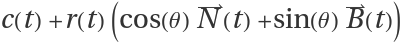, where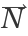and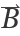are the normal and the binormal vectors of c, respectively.
The radius of the cross section is perpendicular to the tangent of the curve.

## Examples

### Basic Examples (3)

Create tubes from a circle:

 In:=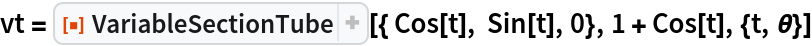Out=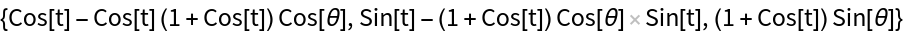Plot them:

 In:=Out=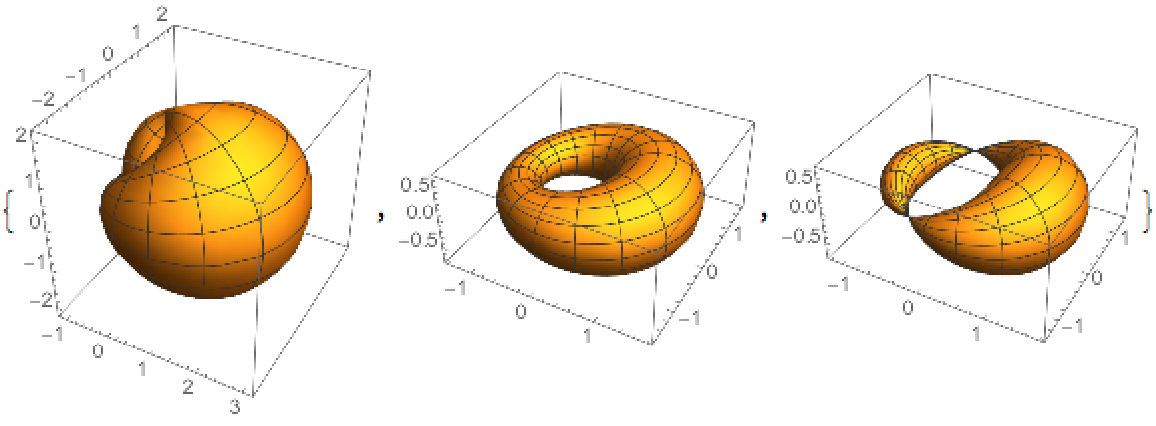Create tubes based on a Sin function with angular frequency 4:

 In:=Out=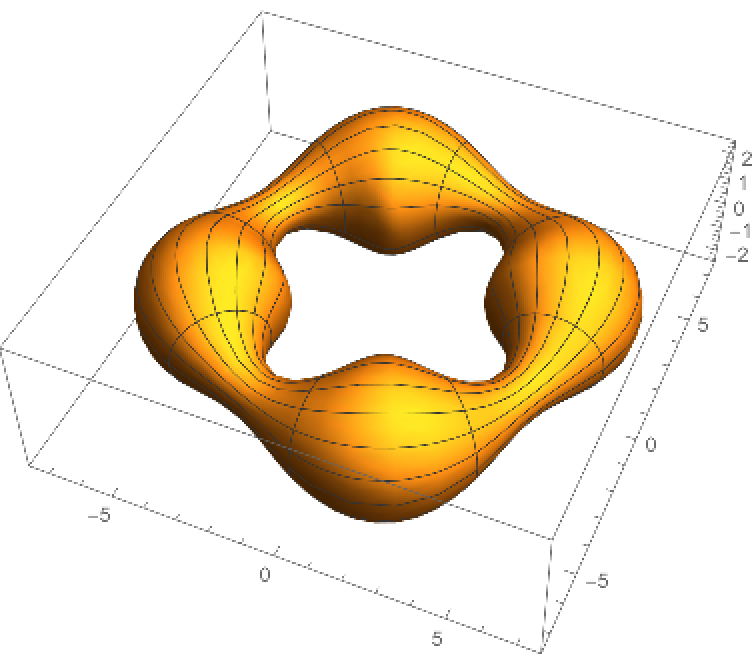Create a tube from a helix:

 In:=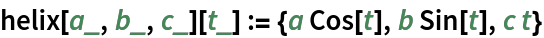In:=Out=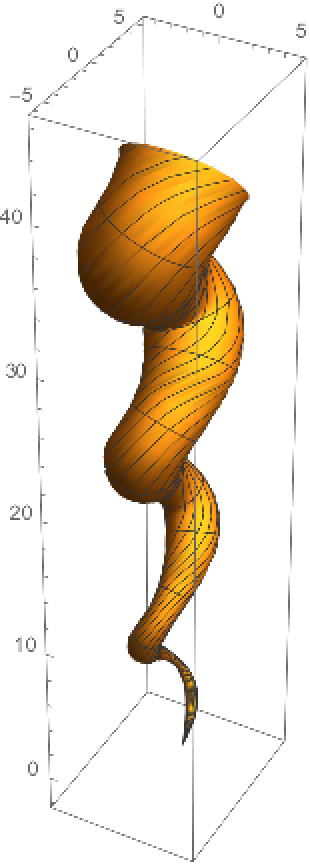Enrique Zeleny

## Version History

• 1.0.0 – 14 July 2020